# 12 Results

View
Selected filters:
• OH.TMM.0005.Core.2Rating

The Calculus I course was developed through the Ohio Department of Higher Education OER Innovation Grant. This work was completed and the course was posted in February 2019. The course is part of the Ohio Transfer Module and is also named TMM005. For more information about credit transfer between Ohio colleges and universities, please visit: www.ohiohighered.org/transfer.Team LeadJim Fowler                                         Ohio State UniversityRita Ralph                                         Columbus State Community CollegeContent ContributorsNela Lakos                                       Ohio State UniversityBart Snapp                                       Ohio State UniversityJames Talamo                                  Ohio State UniversityXiang Yan                                         Edison State Community CollegeLibrarianDaniel Dotson                                    Ohio State University                     Review TeamThomas Needham                             Ohio State UniversityCarl Stitz                                            Lakeland Community CollegeSara Rollo                                          North Central State College

Subject:
Mathematics
Calculus
Material Type:
Full Course
Provider:
Ohio Open Ed Collaborative
09/26/2018Conditional Remix & Share Permitted
CC BY-NC
Rating

After completing this section, students should be able to do the following.Identify word problems as related rates problems.Translate word problems into mathematical equations.Solve related rates word problems.

Subject:
Calculus
Material Type:
Module
Provider:
Ohio Open Ed Collaborative
07/02/2019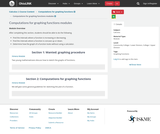Conditional Remix & Share Permitted
CC BY-NC
Rating

After completing this section, students should be able to do the following.Find the intervals where a function is increasing or decreasing.Find the intervals where a function is concave up or down.Determine how the graph of a function looks without using a calculator.

Subject:
Calculus
Material Type:
Module
Provider:
Ohio Open Ed Collaborative
07/02/2019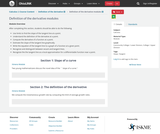Conditional Remix & Share Permitted
CC BY-NC
Rating

After completing this section, students should be able to do the following.Use limits to find the slope of the tangent line at a point.Understand the definition of the derivative at a point.Compute the derivative of a function at a point.Estimate the slope of the tangent line graphically.Write the equation of the tangent line to a graph of a function at a given point.Recognize and distinguish between secant and tangent lines.Recognize the the tangent line as a local approximation for a differentiable function near a point.

Subject:
Calculus
Material Type:
Module
Provider:
Ohio Open Ed Collaborative
06/28/2019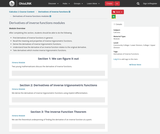Conditional Remix & Share Permitted
CC BY-NC
Rating

After completing this section, students should be able to do the following.Find derivatives of inverse functions in general.Recall the meaning and properties of inverse trigonometric functions.Derive the derivatives of inverse trigonometric functions.Understand how the derivative of an inverse function relates to the original derivative.Take derivatives which involve inverse trigonometric functions.

Subject:
Calculus
Material Type:
Module
Provider:
Ohio Open Ed Collaborative
07/02/2019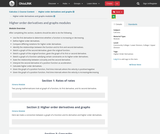Conditional Remix & Share Permitted
CC BY-NC
Rating

After completing this section, students should be able to do the following.Use the first derivative to determine whether a function is increasing or decreasing.Define higher order derivatives.Compare differing notations for higher order derivatives.Identify the relationships between the function and its first and second derivatives.Sketch a graph of the second derivative, given the original function.Sketch a graph of the original function, given the graph of its first or second derivative.Sketch a graph of a function satisfying certain constraints on its higher-order derivatives.State the relationship between concavity and the second derivative.Interpret the second derivative of a position function as acceleration.Calculate higher order derivatives.Given the graph of a position function, find time intervals where the velocity is positive/negativeGiven the graph of a position function, find time intervals where the velocity is increasing/decreasing

Subject:
Calculus
Material Type:
Module
Provider:
Ohio Open Ed Collaborative
06/28/2019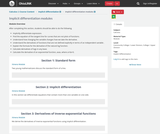Conditional Remix & Share Permitted
CC BY-NC
Rating

After completing this section, students should be able to do the following.Implicitly differentiate expression.Find the equation of the tangent line for curves that are not plots of functions.Understand how changing the variable changes how we take the derivative.Understand the derivatives of functions that are not defined explicitly in terms of an independent variable .Explain the formula for the derivative of the natural log function.Calculate derivatives of logs in any base.Calculate the derivative of an exponential function, axax, where a>0a>0.

Subject:
Calculus
Material Type:
Module
Provider:
Ohio Open Ed Collaborative
07/02/2019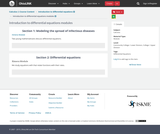Conditional Remix & Share Permitted
CC BY-NC
Rating

Subject:
Calculus
Material Type:
Module
Provider:
Ohio Open Ed Collaborative
07/03/2019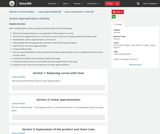Conditional Remix & Share Permitted
CC BY-NC
Rating

After completing this section, students should be able to do the following.Define linear approximation as an application of the tangent to a curve.Find the linear approximation to a function at a point and use it to approximate the function value.Identify when a linear approximation can be used.Label a graph with the appropriate quantities used in linear approximation.Find the error of a linear approximation.Compute differentials.Use the second derivative to discuss whether the linear approximation over or underestimates the actual function value.Contrast the notation and meaning of dydy versus ΔyΔy.Understand that the error shrinks faster than the displacement in the input.Justify the chain rule via the composition of linear approximations.

Subject:
Calculus
Material Type:
Module
Provider:
Ohio Open Ed Collaborative
07/02/2019Conditional Remix & Share Permitted
CC BY-NC
Rating

After completing this section, students should be able to do the following.Identify situations where logs can be used to help find derivatives.Use logarithmic differentiation to simplify taking derivatives.Take derivatives of functions raised to functions.Recognize the difference between a variable in the base and a variable in the exponent.

Subject:
Calculus
Material Type:
Module
Provider:
Ohio Open Ed Collaborative
07/02/2019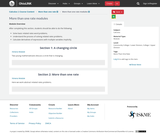Conditional Remix & Share Permitted
CC BY-NC
Rating

After completing this section, students should be able to do the following.Solve basic related rates word problems.Understand the process of solving related rates problems.Calculate derivatives of expressions with multiple variables implicitly.

Subject:
Calculus
Material Type:
Module
Provider:
Ohio Open Ed Collaborative
07/02/2019Unrestricted Use
CC BY
Rating

This is a Calculus I interactive textbook with modules curated and created on The Ohio State University's Ximera platform.

Subject:
Mathematics
Calculus
Material Type:
Textbook
Provider:
Columbus State Community College
Author:
Kevin James
Lee Wayand
Rita Ralph
The Ximera Project
Tzy-Yi "Alan" Yang